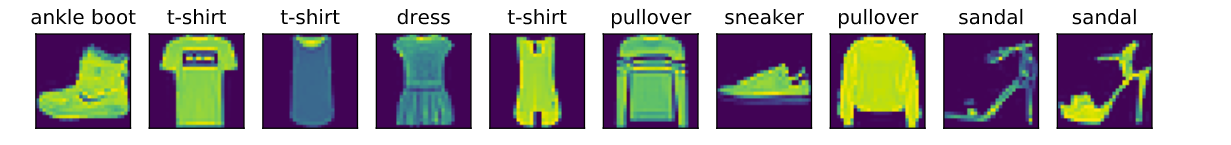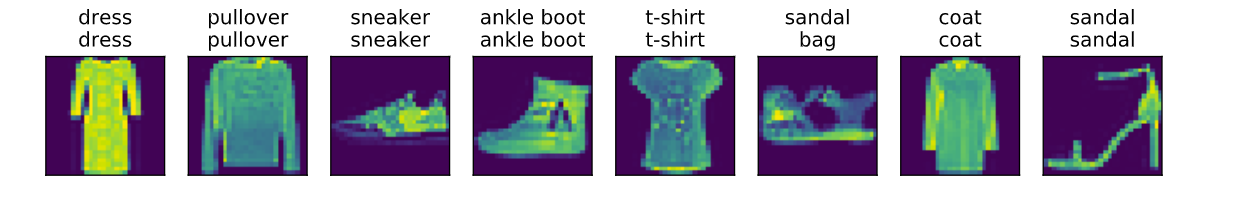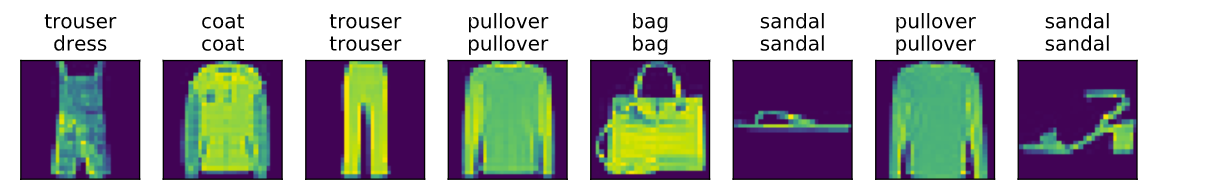# 深度学习学习笔记(3)——softmax回归

本文记录了在学习pytorch版《动手学深度学习》时所涉及到的知识和所做的练习。

# 1 回归与分类

在机器学习中，有监督学习一般可以分为回归和分类两类，回归用来解决标签为连续值的情况，而分类用来解决标签为离散值的情况。比如上一篇文章所解决的房价问题，标签是价格，这就是连续值的情况，所以使用了最简单的线性回归就可以解决。而如果是遇到标签是几个类别的情况，就需要用到分类的方法了。

而softmax回归是线性回归的一种改进方案，虽然它依然叫回归，但是实际上就是用来解决分类问题的，所以是分类的方法。softmax回归用来解决多分类问题，还有一种解决二分类问题的方法也和此类似，叫做逻辑回归。

# 2 softmax回归的简单实现

## 2.1 问题描述

现在有这样一个问题，对于一个图像分类的问题，每次输入2 * 2的像素的数据,分别记为$x_1$，$x_2$，$x_3$，$x_4$，假设真实的标签为鸡、鸭、鱼，分别记为$y_1$，$y_2$，$y_3$。我们先依然按照线性回归的方式来进行建模，这个问题的输入是4个特征，输出则是三个，所以有这样的表达式

如果要让这个模型预测类别，只需要让$y_1,y_2,y_3$三个输出值分别代表三个类别的置信度，这样样本分类的类别就是$y_1$、$y_2$、$y_3$最大值对应的类别。比如最后的结果$y_1=5,y_2=10,y_3=1$，那么这个样本就应该被分为$y_2$对应的鸭。但是这样也存在一个问题，比如有时候输出的$y_1=100$，而$y_2=1,y_3=1$，这样直观上看被分为$y_1$的概率就最大，但是有时候$y_1=100$，而$y_2=10^5,y_3=10^6$，被分为$y_1$的概率就是最小了。所以这样的方法很难确定最后输出的范围，也会导致没有办法衡量最后输出的误差到底是多少。

所以softmax回归就是在这个基础上再加了一个步骤，让所有的输出再经过一个softmax层，再输出最后的结果

其中,

经过softmax层以后，最后输出的$Y_1,Y_2,Y_3$三者的和一定是为1的，并且三个值都在0到1之间，可以把它们理解为该样本分为第i类的概率。比如这个时候$Y_1=0.9$，我们就可以很直观的知道，该样本分为第1类鸡类的概率为0.9，不管其他两个类的概率为多少，我们都可以很轻松的将这个样本分到鸡这个类了。而真实值中，如果该样本的类别为鸡，那么$Y_1$就应该为1,其他的$Y_j$则都为0。

还是像线性回归一样，把所有的表达式都改写为矢量表达式，也便于在代码中实现。其中，令

输出层输出为

那么模型的表达式就可以

## 2.2 交叉熵损失函数

在前面的线性回归中，用到的损失函数是均方误差函数。在实际应用中，不同的问题也要选择不同的损失函数，否则优化的效果会相差非常大，比如说在图像分类的这个问题中，我们应该更关心的是模型预测的分类是否和真实值匹配，而不是关心这个分类的具体概率到底是什么样的，均方误差就会对这个概率要求更加的严格，所以这并不是我们想要的。在softmax回归中，更常用的损失函数是交叉熵损失函数(cross entropy)。

交叉熵定义为

其中：$Y_j^{(i)}$是真实值中第j个类别的值，不是0就是1，而$y_j^{(i)}$就是预测出每个类别的值，在0到1之间，q是类别的总个数。

因为$Y_j^{(i)}$除了真实类别对应的值是为1的，其他都是为0，所以交叉熵其实可以简化为

其中这个下标m是指真实类别对应值为1的这个类别号，因为只有真实值不为1的情况下，乘以0的这部分是没有意义的。

交叉熵那么损失函数就可以写为

其中$\theta$和之前一样，是模型的全部参数。

## 2.3 torchvision数据集

torchvision这个包是服务于PyTorch深度学习框架的，主要用来构建计算机视觉模型。torchvision主要由以下几部分构成：

• torchvision.datasets: 一些加载数据的函数及常用的数据集接口；
• torchvision.models: 包含常用的模型结构（含预训练模型）；
• torchvision.transforms: 常用的图片变换，例如裁剪、旋转等；
• torchvision.utils: 其他的一些有用的方法。

torchvision的datasets里面有很多数据集可以供我们使用，只需要调用torchvision.datasets.数据集名称，就会从网络上把这个数据集下载下来，比如说这个地方我们用到的数据集是FashionMNIST，就调用torchvision.datasets.FashionMNIST，就可以得到训练集和测试集(测试集用来评估最后训练处的模型，防止过拟合)。FashionMNIST是一个装扮类型的数据集，里面都是衣服鞋子之类的图片，而我们就是要根据这些图片，把他们具体属于什么类别给找出来。

但是通过这个方法得到的数据集格式并不是一个tensor，也就不方便使用，所以还要在里面的参数中添加transform=transforms.ToTensor()，将数据转化成tensor。

import torchvision
import torch
import torchvision.transforms as transforms
import matplotlib.pyplot as plt

# 从torchvision的datasets中下载数据集，train=True表示训练集，False表示测试集，再把transform转换成tensor

# 通过索引得到mnist_train的第一个数据，返回的是一个元组，元组的第一个值是特征，第二个则是这个样本的label
feature, label = mnist_train
# 查看这个数据集中数据的shape
print(feature.shape)
print(label)

torch.Size([1, 28, 28])
9

可以看到这个数据集中的特征是[1, 28, 28]这样的形状，其实就是[通道数, 图片的高, 图片的宽]这样的形状，因为这个数据集是灰度图像，所以通道数为1。

FashionMNIST中一共包括了10个类别，分别为t-shirt（T恤）、trouser（裤子）、pullover（套衫）、dress（连衣裙）、coat（外套）、sandal（凉鞋）、shirt（衬衫）、sneaker（运动鞋）、bag（包）和ankle boot（短靴），但是从刚刚输出的label可以看到，其实label是一个数字，所以我们要把数字还要对应成具体的文字类别，才好直观的看到最终的效果。

所以下面就写两个函数，尝试显示出这个数据集中的一些图片来看看。

# 将数据集label对应到具体的类别中
def get_fashionMNIST_label(label):
text_labels = ['t-shirt', 'trouser', 'pullover', 'dress', 'coat',
'sandal', 'shirt', 'sneaker', 'bag', 'ankle boot']
return [text_labels[i] for i in label]

# 显示一排子图，并且打上对应的标签
def show_fashion_mnist(images, labels):
# 在一张图中画多个子图就要用到subplots函数，第一个参数是子图的行数，第二个是子图的列数，figsize是图片的大小，返回值的第一个是画布对象，第二个是子图对象
fig, ax = plt.subplots(1, len(images), figsize=(12, 12))
# 用得到的子图来显示每一张图片，zip就是将这三个变量组成一个元组，方便在for循环中去遍历
for a, img, lbl in zip(ax, images, labels):
# 每一个子图都显示出图片， 这里图片因为是[1,28,28]的形状，把它转换成[28,28]的形状，然后再转成nunpy，如果在gpu上还要先转到cpu上
a.imshow(img.view((28, 28)).cpu().numpy())
# 给子图显示一个标题，也就是标签名
a.set_title(lbl)
# 把每一个子图的坐标x和y轴都设置为不可见
a.axes.get_xaxis().set_visible(False)
a.axes.get_yaxis().set_visible(False)
plt.show()

X, y = [], []
# 从训练集中得到十个图片和标签
for i in range(10):
X.append(mnist_train[i])
y.append(mnist_train[i])
# 调用函数显示图片
show_fashion_mnist(X, get_fashionMNIST_label(y))import torch.utils.data as data
lr = 0.05
BATCH_SIZE = 256
NUM_EPOCH = 10
device = 'cuda' if torch.cuda.is_available() else 'cpu'
NUM_INPUT = 784
NUM_OUTPUT = 10

test_iter = data.DataLoader(mnist_test, batch_size=BATCH_SIZE, shuffle=True)

## 2.4 实现softmax回归模型

还是像之前一样，先不用pytorch的框架来实现模型，和之前的模型中，主要的不同就是在线性回归的输出后面加一个softmax操作，所以这里先把softmax所做的操作定义为一个函数。

然后还有一个不一样的地方是损失函数，这里用的是交叉熵损失函数，所以下面也要自己实现损失函数的计算，其他的地方比如回归、优化算法，都是采用和线性回归一样的方法。

在初始化模型参数的时候要注意，因为上面问题描述中，$2\star2=4$的输入，每一个标签的输出都需要4个权重，而上面一共有3个标签所以最后的权重参数是一个$4 \star 3$的矩阵，而在这个数据集中，一共是$28 \star 28=784$的输入，一共有10个类别，所以这个地方权重参数应该是一个$784 \star 10$的矩阵，偏差参数也就是$1 \star 10$的矩阵。

# 初始化模型参数
w = torch.zeros(784, 10, device=device, requires_grad=True)
b = torch.zeros(1, 10, device=device, requires_grad=True)

# 具体计算公式见上面的softmax运算公式
def softmax(y):
# 取指数
y_exp = y.exp()
# sum函数是求和，其中的参数dim=0是对列求和，dim=1是对行求和keepdim=True是求和以后保留行和列的维度，为false的话求和以后就只有一个维度了
y_exp_sum = y_exp.sum(dim=1, keepdim=True)
return y_exp / y_exp_sum

# 具体计算公式见上面的模型表达式
def net(X):
# 把X变成1*784的矩阵，然后和权重参数做矩阵乘法，再加上偏差
y = X.view(-1, NUM_INPUT) @ w + b
return softmax(y)

在实现损失函数之前，先了解一下pytorch的gather函数，这个函数可以根据给定的索引，从tensor中取出具体的值出来，比如说，我们预测两个样本，输出分别为[0.3, 0.4, 0.3]和[0.2, 0.3, 0.5]，说明了第一个样本应该被分为第二类，第二个样本应该被分为第三类。而这两个样本真实的label值分别为2,3，我们现在就要根据这个输出和真实值进行比较，计算出交叉熵损失函数。刚好前面描述损失函数时，说到了其实这个交叉熵主要关心的就是这个样本真实类别的预测概率(因为其他都是乘以0)，所以这个gather函数，就能根据这个2和3，分别从输出中取出第二个索引和第三个索引，来计算最后的损失值。
# gather函数使用例子：假设有两个样本，预测概率分别为[0.3, 0.4, 0.3]，[0.2, 0.3, 0.5]
y_pred = torch.tensor([[0.3, 0.4, 0.3], [0.2, 0.3, 0.5]])
# 样本的真实类别为第2类和第3类，具体值因为是从0开始计数，所以是1和2
y = torch.tensor([1,2])
# gather的第一个参数是维度，1就是行，0就是列，第二个参数y是根据这个y里面的值，在对应的行中取索引
# 也就是y的第一行是2，那么y_pred的第一行就取索引为2的数，y的第二行是3，那么y_pred的第二行就取索引为3的数，所以要先把y的shape从1*2改为2*1
x = y_pred.gather(1,y.view(-1,1))
# 取出来的概率就应该是0.4和0.5
print(x)

# 具体计算公式见上面的优化版交叉熵损失函数
def crossEntropy(y_pred, y):
return -1 * torch.log(y_pred.gather(1,y.view(-1,1)))

tensor([[0.4000],
[0.5000]])

除了交叉熵损失函数，在分类问题中还可以用准确率来评估分类的效果，准确度一般都用在测试集或者验证集中来评估模型。计算方法也很简单，把预测正确的个数除以预测的总数就得到准确率了。
# 评估测试集的准确率
def accuracy(data_iter, net):
acc_num = 0
total_num = 0
for X, y in data_iter:
X = X.cuda()
y = y.cuda()
# 得到输出预测值
y_pred = net(X)
# argmax可以得到最大的值的索引值，dim=1就是在行上面找最大值，dim=0就是在列上面找
acc_num += (y_pred.argmax(dim=1) == y).float().sum().item()
# 预测的样本总数
total_num += y.shape
return acc_num / total_num

# 可以先测试一下准确率，因为模型和参数都已经实现完成，只是还没有训练的，所以现在的准确率肯定会很低
print(accuracy(test_iter, net))

0.1

接下来就是训练模型的实现，其中优化器还是使用上一个线性回归中实现的sgd优化函数。
# 定义参数迭代的优化算法
def sgd(params, learning_rate, batch_size):
for param in params:
param.data = param.data - learning_rate * param.grad / batch_size

loss_fn = crossEntropy

for epoch in range(NUM_EPOCH):
# 这三个参数分别是训练集损失值，训练集准确度，总训练样本, 每一个epoch的准确度
train_loss, train_acc, total_num = 0, 0, 0

for X, y in train_iter:
# 把训练数据放到gpu上
X = X.cuda()
y = y.cuda()
# 得到预测值
y_pred = net(X)
# 根绝预测值和真实值得到损失值
loss = loss_fn(y_pred, y).sum()
# 自动求导
loss.backward()
# sgd优化算法
sgd([w,b], lr, BATCH_SIZE)
# 要记得梯度清零

train_loss += loss.item()
train_acc += (y_pred.argmax(dim=1) == y).float().sum().item()
total_num += y.shape
test_acc = accuracy(test_iter, net)
print('当前第{}轮 训练集：loss = {:.4f}, acc = {:.4f}  测试集：acc = {:.4f}'.format(epoch+1, train_loss/total_num, train_acc/total_num, test_acc))

当前第1轮 训练集：loss = 0.9111, acc = 0.7238  测试集：acc = 0.7675

模型训练完成后，用这个模型来对测试集做一些预测，来直观的感受一下模型的效果。
# 得到测试集的一个batch的数据,，先把test_iter转换成diedaiq，然后迭代器需要用next来返回下一个迭代器的数据，因为shuflle过，所以每一次数据都不一样
X, y = iter(test_iter).next()
X = X.cuda()
y = y.cuda()

# 得到真实的类别
true_labels = get_fashionMNIST_label(y.cpu().numpy())
# 得到预测的的类别
pred_labels = get_fashionMNIST_label(net(X).argmax(dim=1).cpu().numpy())
# 把得到的真实类别和预测类别组成新的标签，上面是真实类别，下边是预测类别，绘图的时候显示的标题就是这个组合后的标签
titles = [true + '\n' + pred for true, pred in zip(true_labels, pred_labels)]
# 绘图函数，只绘制前8个数据
show_fashion_mnist(X[0:8], titles[0:8])可以看到，对于大多数的图片都能很好的预测正确了，但是还有一部分图片无法识别出来。

# 3 pytorch版softmax回归

在简单实现了softmax以后，再使用pytorch框架来实现softmax，还是和之前一样，首先要把数据集读取下来。然后再使用nn来构建softmax模型。

import torchvision
import torch
import torchvision.transforms as transforms
import matplotlib.pyplot as plt
import torch.utils.data as data
# 设置超参数
lr = 0.05
BATCH_SIZE = 256
NUM_EPOCH = 10

# 输入的维度和输出的维度
NUM_INPUT = 784
NUM_OUTPUT = 10

# 从torchvision的datasets中下载数据集，train=True表示训练集，False表示测试集，再把transform转换成tensor

test_iter = data.DataLoader(mnist_test, batch_size=BATCH_SIZE, shuffle=True)

具体模型的构建和上一节的线性回归是基本一样的，只是要注意一点，我们得到的数据的形状是[通道数, 图片的高, 图片的宽]这样的形状，然后我们又给数据分成了很多个batch，所以实际输入的形状应该是[batch_size, 通道数, 图片的高, 图片的宽]，所以我们还要这个形状进行转换，转换成[batch_size, NUM_INPUT]才能作为模型输入。

并且pytorch提供了交叉熵损失函数，可以直接调用。

import torch.nn as nn
import torch.optim
import torch.nn.init as init

class LinearNet(nn.Module):
def __init__(self, NUM_INPUT, NUM_OUTPUT):
super(LinearNet, self).__init__()
self.linear = nn.Linear(NUM_INPUT, NUM_OUTPUT)

def forward(self, x):
# 输入的x的shape是[batch_size, 1, 28, 28]
# 要把它的shape变成[batch_size, NUM_INPUT], x.shape就是x在第一维度的size，也就是batch_size
return self.linear(x.view(x.shape, -1))

net = LinearNet(NUM_INPUT, NUM_OUTPUT)
net.cuda()
# 初始化参数, 有时候对参数的不同初始化，也会决定最后训练出来模型的效果
init.normal_(net.linear.weight, mean=0, std=0.01)
init.constant_(net.linear.bias, val=0)

# pytorch提供的交叉熵损失函数
loss_fn = nn.CrossEntropyLoss()
optimizer = torch.optim.SGD(net.parameters(), lr=lr)

for epoch in range(NUM_EPOCH):
# 这三个参数分别是训练集损失值，训练集准确度，总训练样本, 每一个epoch的准确度
train_loss, train_acc, total_num = 0, 0, 0

for X, y in train_iter:
X = X.cuda()
y = y.cuda()
y_pred = net(X)
loss = loss_fn(y_pred, y)
# 把梯度清零
loss.backward()
optimizer.step()

train_loss += loss.item()
train_acc += (y_pred.argmax(dim=1) == y).float().sum().item()
total_num += y.shape

test_acc = accuracy(test_iter, net)
print('当前第{}轮 训练集：loss = {:.4f}, acc = {:.4f}  测试集：acc = {:.4f}'.format(epoch+1, train_loss/total_num, train_acc/total_num, test_acc))

当前第1轮 训练集：loss = 0.0036, acc = 0.7252  测试集：acc = 0.7700

同样在模型训练完成以后，对测试集的图片进行分类，来直观的感受一些模型的效果。
# 得到测试集的一个batch的数据,，先把test_iter转换成diedaiq，然后迭代器需要用next来返回下一个迭代器的数据，因为shuflle过，所以每一次数据都不一样
X, y = iter(test_iter).next()
X = X.cuda()
y = y.cuda()

# 得到真实的类别
true_labels = get_fashionMNIST_label(y.cpu().numpy())
# 得到预测的的类别
pred_labels = get_fashionMNIST_label(net(X).argmax(dim=1).cpu().numpy())
# 把得到的真实类别和预测类别组成新的标签，上面是真实类别，下边是预测类别，绘图的时候显示的标题就是这个组合后的标签
titles = [true + '\n' + pred for true, pred in zip(true_labels, pred_labels)]
# 绘图函数，只绘制前8个数据
show_fashion_mnist(X[0:8], titles[0:8])# 4 总结

• softmax回归名字虽然叫回归，但是其实是解决分类问题的方法，它使用softmax运算输出类别的概率分布。
• softmax回归是一个单层神经网络，输出个数等于分类问题中的类别个数。
• 交叉熵损失函数适合衡量两个概率分布的差异。